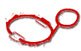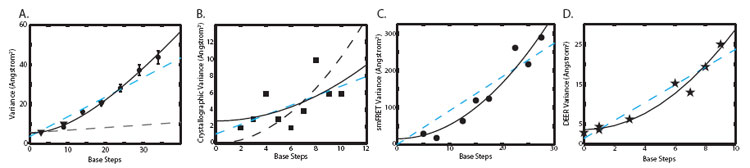SSRL Science Highlight - December 2008The first crystal structures of DNA double helices appeared in 1979 and directly confirmed the atomic model of Watson-Crick from 25 years earlier. Since then, DNA has been modeled as a relatively rigid polymer. An isotropic elastic rod model of the polymer has proven to be exceptionally good at integrating classical biochemical measurements on DNA with recent single-molecule results for DNA on length scales of 100 nm or longer. Nevertheless, unusual mechanical properties have been reported recently with regard to twist-stretch coupling  and bending rigidity [2-3]. Still missing, however, has been a direct measurement of DNA structural fluctuations on short length scales in the absence of external force.

In principle, molecular rulers should be ideal tools for characterizing DNA structural fluctuations. Spectroscopic molecular rulers are frequently used to measure distances in solution. Existing techniques suffer from two limitations: they do not provide quantitatively accurate distance values and they cannot accurately determine distributions composed of multiple different distances. An alternative physical phenomenon on which to base a molecular ruler is X-ray scattering interference. Solution X-ray scattering techniques are primarily used to obtain the average compactness of macromolecules. To allow for site-specific measurements, one could incorporate strongly-scattering probes into a structure and detect the scattering interference between them. Fourier transformation of this interference signal would directly yield the distribution of interprobe distances.

In this work, we have developed a molecular ruler that utilizes 14-Å gold nanocrystal probes attached site-specifically to DNA duplexes. The experiments were carried out at beamline 4-2 of the Stanford Synchrotron Radiation Lab (SSRL). We measured the pattern of X-ray scattering interference between the nanocrystals and transformed it into the point-to-point distance probability distribution between their centers-of-mass (Figure 1). To determine the scattering interference pattern between two gold nanocrystals attached to a DNA duplex, five different scattering profiles were collected. Profiles for the gold nanocrystals alone, DNA alone, two single-labeled DNA duplexes and a double-labeled DNA duplex were acquired and scaled relative to each other, then summed to generate a nanocrystal interference pattern (Figure 1A). These patterns were transformed into inter-nanocrystal center-of-mass distance distributions using a non-negative least squares algorithm (Figure 1B).Figure 1: [A] Scattering intensity as a function of scattering angle for the 20 base-pair double-labeled (blue), single-labeled (red, magenta; indistinguishable), and unlabeled (cyan) DNA duplexes. The intensity of the double-labeled sample has been scaled by a factor of 1/2 to aid visual comparison. The pattern of scattering interference between the two nanocrystal labels (black) is obtained by summing the intensities of the double-labeled and unlabeled samples, then subtracting the intensities of the two single-labeled samples. [B] Transformation of the nanocrystal scattering interference pattern into a weighted sum of sinusoidal basis functions (corresponding to different interprobe distances) yields the probability distribution for nanocrystal center-of-mass separation.Figure 2: Probability distance distribution curves for the 10-bp (red), 15-bp (green), 20-bp (black), 25-bp (cyan), 30-bp (magenta), and 35-bp (blue) duplexes. The distributions were normalized to sum to unity. Each distribution was fit to a Gaussian curve (yellow).
Scattering interference profiles for DNA duplexes ranging from 10 to 35 base-pairs in length were measured and gave distance distributions with approximately symmetric fluctuations around a well-defined mean distance (Figure 2). The interprobe distances were fit to a three variable model of the DNA helix and indicate a helical rise value of 3.29 � 0.07 Å, in close agreement with the crystallographic average value of 3.32 � 0.19 Å . Structural fluctuations of the DNA should be reflected in the width of the measured distance distributions after DNA-independent factors have been taken into account (Figure 3). The distance distributions are not consistent with the conventional model of the DNA duplex as an isotropic elastic rod with a stretch modulus of ~1000 pN. A linear fit of the observed variances with respect to DNA length indicates an apparent stretch modulus of ~133 pN. The elastic rod model predicts that the variance should increase linearly with the number of base steps (Figure 3A). In contrast, we observe a partial quadratic dependence of variance on DNA length. The data fit a partial quadratic dependence to within the measurement error (black line; X2 = 7.5 with 6 degrees of freedom; P = 0.28), but not a linear dependence (cyan dashed line; X2 = 41 with 7 degrees of freedom; P = 1.0 x 10-17). A quadratic increase in variance can only occur if the stretching fluctuations are tightly correlated. Fits to models that interpolate between linear and quadratic dependences with a range of correlation lengths demonstrate that the stretching correlation must persist over two turns of the double helix. The data indicate that short DNA fragments stretch cooperatively.

In light of this surprising observation, we reexamined previous structural studies of short DNA duplexes. Analysis of the end-to-end lengths for DNA duplexes in the Nucleic Acid Database revealed a range of distances that is consistent with our solution observations. However, a plot of crystallographic length variance with respect to the number of base steps is inconclusive with respect to the cooperativity of DNA stretching (Figure 3B). Plots of the end-to-end length variance derived from time-resolved single molecule FRET and electron spin resonance data with respect to duplex length are better fit by a quadratic relationship than by a linear relationship (Figure 3C-D). These independent measurements support the conclusion that short DNA duplexes stretch cooperatively.

These findings, taken together with other recently discovered nonideal properties of DNA [1-3], imply that a new theoretical framework is required to describe the mechanical properties of DNA on short length scales. The presence of stretching correlations over 20 base pairs implies that DNA double helices can transmit information through an allosteric "domino effect". Whether such allosteric communication alters how the double helix and its specific binding partners interact to regulate biological processes remains to be seen but is an exciting possibility.Figure 3: [A] Variance in nanocrystal-nanocrystal separation distance of end-labeled duplexes (circles) and internally labeled duplexes (triangles), plotted with respect to the number of intervening DNA base-pair steps. The variance predictions for an ideal elastic rod with a stretching modulus of 1000 pN (the value measured in single-molecule stretching experiments) are shown (dashed black line) and deviate grossly from the data. A linear relationship between variance and base-pair steps (dashed cyan line) is expected if the stretching of base-pair steps is uncorrelated along the DNA duplex. Alternatively, a partial quadratic relationship (solid black line) should hold if the DNA stretches cooperatively with a correlation length of 20 base-pair steps. [B] Variance of the inter-strand separation distance between 3'-phosphates as measured by X-ray crystallography [squares] is plotted as a function of the number of intervening base-steps. [C] Variance of the inter-strand separation distance between fluorophores as measured by time-resolved single-molecule FRET (trsmFRET) experiments  [circles] is plotted as a function of the number of intervening base-steps. [D] Variance of the inter-strand separation distance between nitroxide labels as measured by double electron-electron resonance (DEER) experiments  (click here for higher resolution image)

Primary Citation:
R.S. Mathew-Fenn, R. Das, J.A. Silverman, P.A. Walker, P.A.B. Harbury, "A Molecular Ruler for Measuring Quantitative Distance Distributions." PLoS ONE 3,e3229 (2008).

R.S. Mathew-Fenn, R. Das, P.A.B. Harbury, "Remeasuring the Double Helix." Science 322, 446 (2008).

References:

 J. Gore et al., Nature 442, 836 (2006). T.E. Cloutier, J. Widom, Mol. Cell 14, 355 (2004). T.E. Cloutier, J. Widom, Proc. Natl. Acad. Sci. USA 102, 3645 (2005). W.K. Olson, A.A. Gorin, X.J. Lu, L.M. Hock, V.B. Zhurkin, Proc. Natl. Acad. Sci. USA 95, 11163 (1998). T.A. Laurence, X. Kong, M. Jager, S. Weiss, Proc. Natl. Acad. Sci. USA 102, 17348 (2005). Q. Cai et al., Nucleic Acids Res. 34, 4722 (2006).

| PDF version | | Lay Summary | | Highlights Archive |

SSRL is supported by the Department of Energy, Office of Basic Energy Sciences. The SSRL Structural Molecular Biology Program is supported by the Department of Energy, Office of Biological and Environmental Research, and by the National Institutes of Health, National Center for Research Resources, Biomedical Technology Program, and the National Institute of General Medical Sciences.

 Last Updated: 20 November 2008 Content Owner: R.S. Mathew-Fenn and P.A.B. Harbury, Stanford University Page Editor: L. Dunn